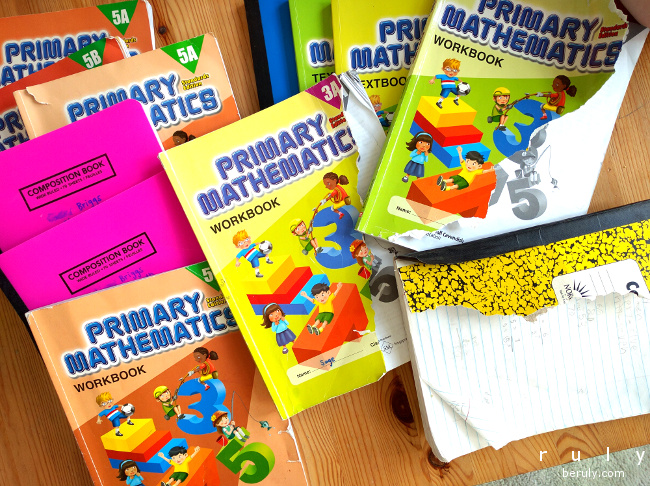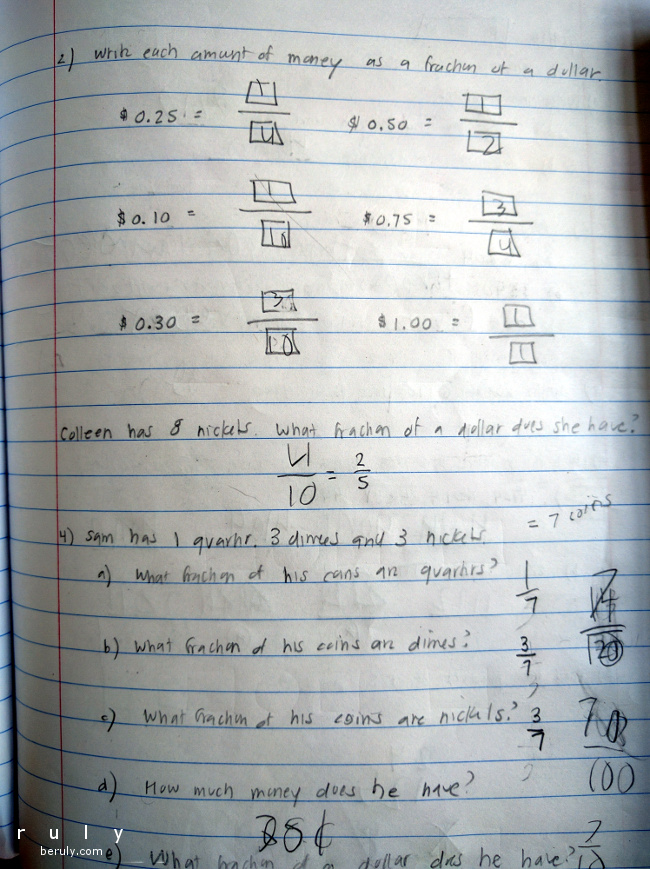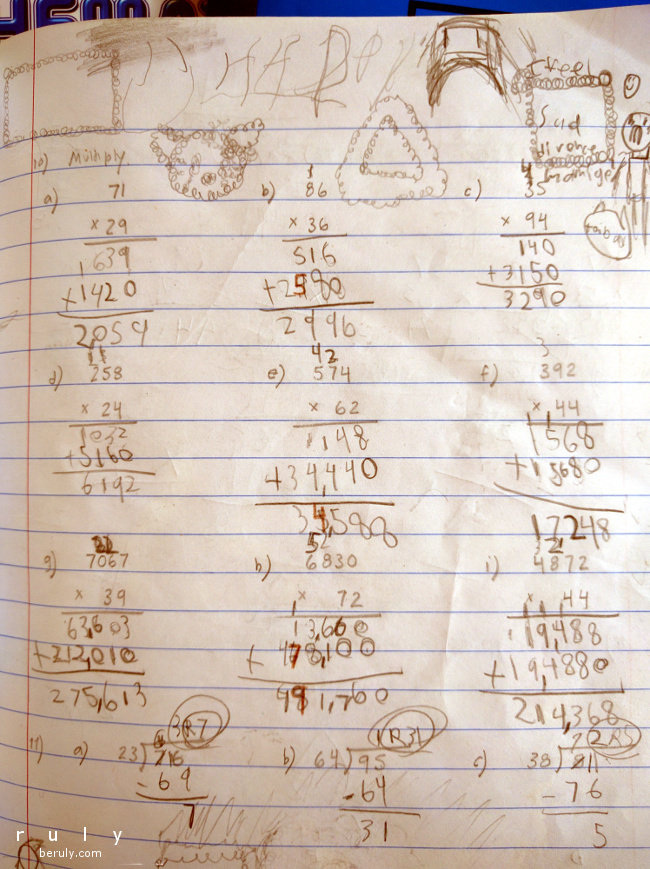# We Finished Math!Despite all of the messy bits of March, including a monster cold that has overtaken all of us this week, we can claim victory on one to do list item . . . . WE FINISHED MATH!!!

For us, math is the most time consuming part of our homeschool schedule. The curriculum we are using has to be taught individually to each child. My eldest does 5th grade math, my second daughter completed 3rd grade math and my preschooler is making progress on his Pre-K/kindergarten math.

In general, the math curriculum is the one we struggle the most to finish each year. It is always a dash to the finish in June, with a lot of crunch time at the end. It is so stressful. Up until I got sick with flu and pneumonia in November, we had been doing so well to stay on track with the math. Then we didn’t do math for almost a month! When December rolled around, we doubled up and did twice the lessons each day so that we could finish the first half by Christmas and even proudly displayed our completed math books for Santa.

After Christmas, we had ski class to look forward to on Fridays so we knew we were going to miss math at least once a week. So, we kept going with the two lessons a day. There was often a lot of grumbling but here we are at the end of March ALL DONE!

Fifth grade math was the first year where math began to get hard for me as a teacher. There were several story problems I had to Google to find out how to solve them. Fortunately, many other parents had the same problem and there are many supremely mathematically skilled people on the Internet who are willing to give answers and excellent explanations! Singapore Math likes to throw in tricks from time to time and the problems I was getting stuck on were usually of the type where you thought you didn’t have enough information to solve the problem until you saw some hidden trick or pattern. People who are great at math have genius skills in seeing hidden patterns.

As a quick summary of what we learned this year.

3rd Grade Math = 681 pages plus 1 ½ notebooks worth of scratch paper

• Multiplying up to the 10 times tables (I have noticed this is a huge difference between US and foreign math curricula. In the US we tend to go up to 12 because we often need to know 12 times when we measure in feet. In other countries, 12 is less important, because their measurement systems are based on multiples of 10, so going up to 10 is enough. They have tricks for multiplying above 10 that they use instead of memorizing the 11 and 12 times tables.)
• Adding, Subtracting, Multiplying and Dividing Numbers in the Thousands
• Long division
• Measurements in US and International Units (inches, feet, yards, cm, m, kg, lb, oz, etc.)
• Money – Adding, subtracting, multiplying and dividing
• Fractions
• Telling time to the minute
• Geometry – identifying right angles, calculating area, perimeter and volume
• Story problems may require as many as 4 stepsOne organizing trick for homeschool math papers that only took me about 4 years to learn is that if you can do all of the assignments, teaching and scratch paper in one composition book, you have a lot fewer papers to keep track of. So, it takes a little time to copy down the math problems into the notebook but in the end makes a clean, neat record that is easy to store.

5th Grade Math – 621 pages! Plus 1 ½ notebooks worth of scratch paper

• Multiplying and dividing by 2-digit numbers
• Adding, subtracting, *multiplying and *dividing fractions
• Order of operations – i.e. parentheses first, then multiplication and division left to right then addition and subtraction left to right
• Perimeter, Area, Volume
• Equivalent Ratios
• Adding, subtracting, multiplying and dividing decimals
• *Converting fractions to percentages
• *Geometry – finding unknown angles in complex diagrams using principles of isosceles and equilateral triangles, parallelograms, rhombuses and trapezoids
• Calculating averages – finding average, median and mode
• Interpreting pie charts and line graphs
• *Beginning Algebraic expressions, graphing equations
• Story problems may require numerous steps, sometimes as many as 7 calculations!

*indicates concepts not taught in our local school system for 5th gradeThe scratch paper also serves as a medium of expression for boredom and frustration!

The geometry and algebra in the fifth grade stunned me. My husband and I didn’t learn any of this until about 7th grade or so. I checked around with other smart parents and they say the same. We are pushing down so much material to the younger grades. My kids so far are handling it well but I consider them “above average” (a la Lake Wobegon). I hope there aren’t too many children out there who think they are no good at math when really they might just do better on the older, more relaxed schedule.

When I compare the curriculum we are using to the concepts taught in our local school district, it seems we map fairly closely up until the end of 3rd grade. Beginning in the 4th grade, Singapore Math seems to push about 1 grade higher than the school district. Many of the concepts listed for 5th grade math we covered in 4th grade.

I read an article recently praising the Finnish mathematical system for being less pressured. Yet, when I cross-referenced the Finnish standards with what we learned they seemed to be right on track with us for 5th grade math. So, perhaps the Finns slow down sometime after 5th grade but up until then I wouldn’t call their math education programs “relaxed.”

So, what now that we are “finished” with math? We will keep reviewing some things until we take our standardized tests for the year but we are moving on to focus on a kids JavaScript computer programming book. I know nothing about JavaScript but I am learning as we go.